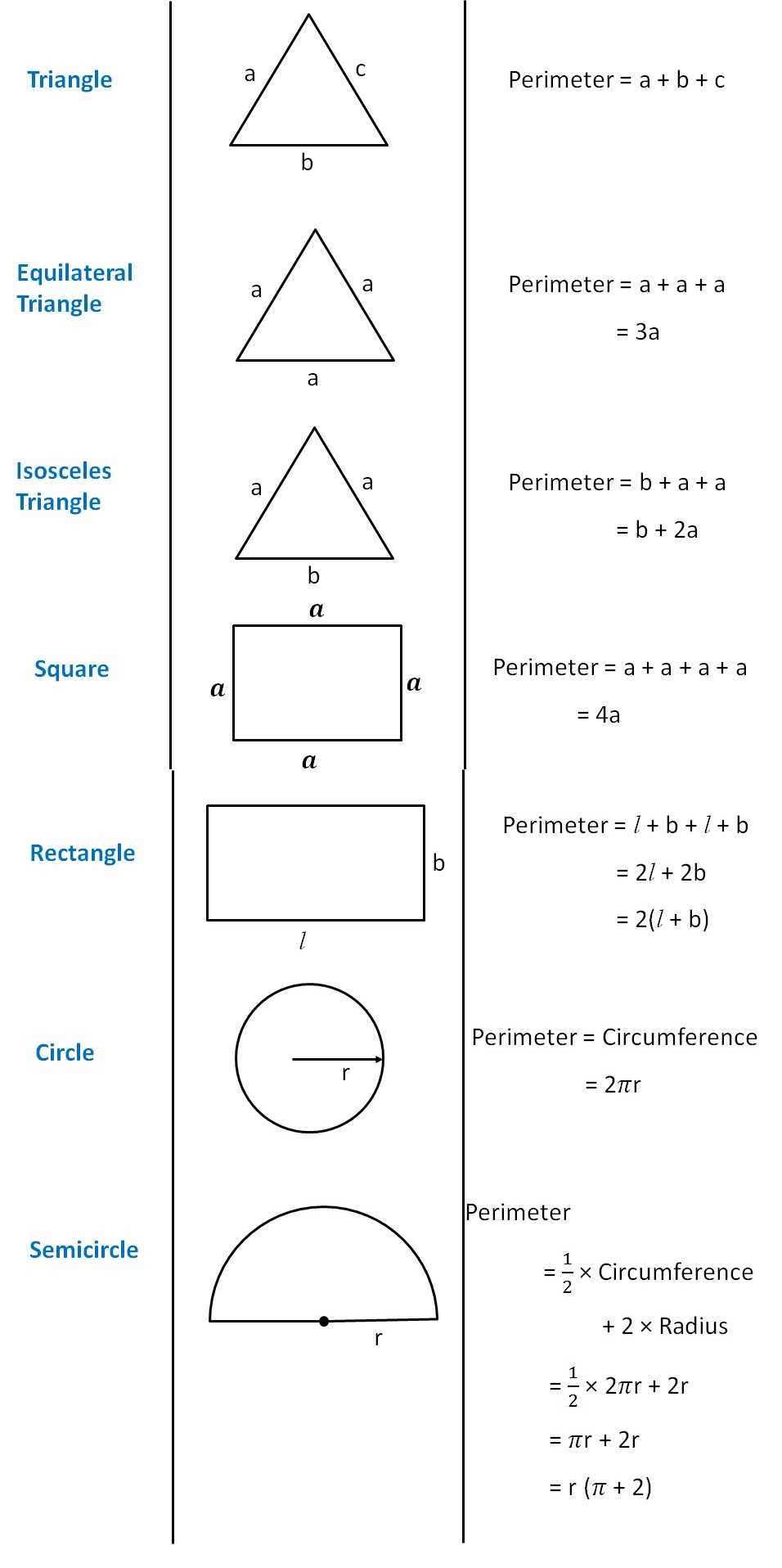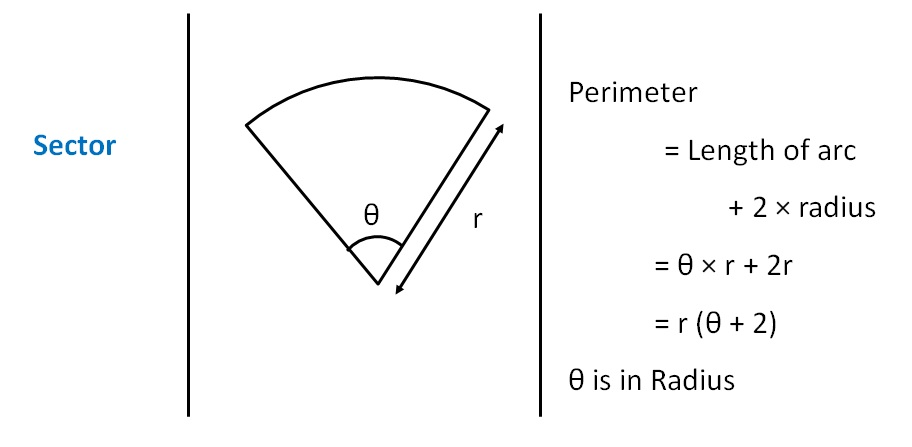Perimeter as variable

Chapter 11 Class 6 Algebra
Concept wise

Perimeter is the length of outline of the shape

For some shapes,

Perimeter = Sum of all sides

Let’s look at the perimeter of common shapes